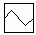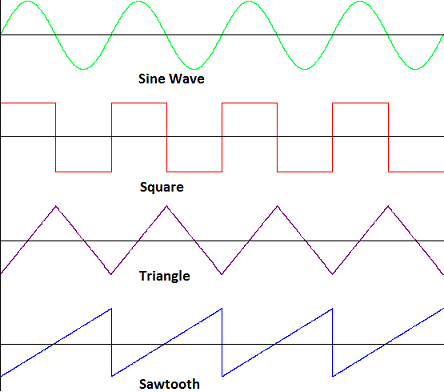# Controller -- Waveform Controller

Jump to navigation Jump to search

Navigation: Main Page -> Models -> Control 2 Models

This model is only available in SysCAD 9.3 Build 136 and later versions.

## General Description

• This unit operation is used to simulate process variable variation based on waveform. It can be used to add disturbance to Feeders, pipe outlet capacity and other writeable variables.
• The Waveform Control model is an orphan model, i.e it cannot be connected to any other unit operation.
• The Waveform model is for dynamic simulation only with the purpose of adding disturbance to the model.

### DiagramThe diagram shows the default drawing of the waveform control unit.

## Inputs and Outputs

There are no connections to this unit.

## Model Theory

The waveform generation have the following 4 forms:

1. Sine Wave
2. Square Wave
3. Triangle Wave
4. Sawtooth Wave### Sinewave function

$y(t) = (Max - Centre) + \frac{PeakToPeak}{2} * sin(\frac{2\pi * (t + TimeOffSet)}{Period})$
where
Max = Maximum value
Centre = Mid point of the Peak to Peak value = (Maximum value - Minimum Value)/2.
PeakToPeak = Peak to Peak value on the y scale, Maximum value - Minimum Value.
Timeoffset = Starting point (time) of wave, if Timeoffset = 0, then the sine wave will start at centre value.
t = time, each iteration t = t + step size.
Period = 1/Frequency, time taken for one full wave cycle.

For example

If the min feed is 10 t/h and the Max feed is 50 t/h, using a Period of 1 hour with no time offset, the sine wave form will generate the feed input curve as per left image below.

If the time offset is 900 seconds (1/4 of the total period), then the sine wave is shifted by 1/4 cycle, as per the right image below:

## Data Sections

The default sections and variable names are described in detail in the following tables. The default Waveform Controller access window consists of 3 sections. This number may increase or decrease, based on user configuration.

### Summary of Data Sections

1. SignalCon tab - Contains a summary of all of the individual Waveform Controls contained in the unit.
2. SW Tabs tab - This page contains all of the information for each individual Waveform Controls, starting at 1 for the first Waveform Controls.
3. Info tab - Contains general settings for the unit and allows the user to include documentation about the unit and create Hyperlinks to external documents.

### SignalCon

Unit Type: SignalCon - The first tab page in the access window will have this name.

 Tag / Symbol Input/ Calc Description On Tick Box The Overall Waveform Control unit will be enabled or disabled using this box. This means that all of the independent Waveform controllers in the unit will be disabled. ShowCnv Tick Box With this option selected, SysCAD will display engineering units for the Output tag. Note that these will only be displayed after SysCAD has completed at least one iteration. Count Input The number of independent Waveform controllers required. This may be any number from 1 upwards. The user may also change this number at any time. The unit will always add new Waveform controllers after existing ones. User may delete individual Waveform using the 'Delete Me' button under the individual Waveform controller blocks. SetAtStartUp Tick Box With this option selected, the waveform controller output tags will be set during Startup step. CommonPeriod Tickbox If this option is selected, then all the individual waveform controller blocks in the current unit will use the same Time period and offset. TimePeriod Input Only visible if the CommonPeriod option is selected, all the individual waveform controller blocks in the current unit will use the same Time period. Frequency Calc The frequency of the cycle. The reciprocal of TimePeriod. Frequency = 1 / TimePeriod. TimeOffset Input Only visible if the CommonPeriod option is selected, all the individual waveform controller blocks in the current unit will use the same offset. Check Tags Button SysCAD will perform a check on the validity of the tags and functions used in the waveform controllers. Summary shows a summary table with the following values for each individual waveform controller in the unit. SW Display The waveform Controller number Action On The waveform generator is on and the controller will be setting the output tag. Off The waveform generator is off and the controller will NOT be setting the output tag. Manual (User) The waveform generator is off but the controller will be setting the output tag to the User value (ManualOutput). Manual (Min) The waveform generator is off but the controller will be setting the output tag to MinValue. Manual (Max) The waveform generator is off but the controller will be setting the output tag to MaxValue. Manual (Centre) The waveform generator is off but the controller will be setting the output tag to the Centre value ((MaxValue-MinValue)/2). Output Display The output value for the individual waveform Controller. OutputUnitTag Display The Unit Operation Tag for each individual waveform Controller. For example: If the Output Tag is Plant_Feed.QmReqd (t/h), then the OutputUnitTag is Plant_Feed.

### SW1: Individual Signal Waveform Controller Data Fields

Individual Waveform Controllers are displayed on the SWx pages. Each page displays up to four Waveform Controllers.

 Tag / Symbol Input/ Calc Description Cfg.[SW number] Action On The waveform generator is on and the controller will be setting the output tag. Off The waveform generator is off and the controller will NOT be setting the output tag. Manual (User) The waveform generator is off but the controller will be setting the output tag to the User value (ManualOutput). Manual (Min) The waveform generator is off but the controller will be setting the output tag to MinValue. Manual (Max) The waveform generator is off but the controller will be setting the output tag to MaxValue. Manual (Centre) The waveform generator is off but the controller will be setting the output tag to the Centre value ((MaxValue-MinValue)/2). Name Input The user may give the waveform Controller a name that describes the control, such Feed_Variation. WaveformType Sine Waveform that starts from the midpoint value, then completes one sine wave. See Model Theory. NOTE the starting point might be shifted by the TimeOffset value. Square Waveform that uses the Minimum value for 1/2 cycle then the Maximum Value for 1/2 cycle to complete one full wave cycle. NOTE the starting point might be shifted by the TimeOffset value. Triangle Waveform that starts from the Minimum value, then linearly increases to the Maximum value, thereafter linearly reduces to the Minimum valve to complete one full wave cycle. NOTE the starting point might be shifted by the TimeOffset value. Sawtooth Waveform that starts from the Minimum value, then linearly increases to the Maximum value to complete one full wave cycle. NOTE the starting point might be shifted by the TimeOffset value. ManualOutput Input Only visible when Action is set to Manual (User). The output tag will be set to this value instead of a waveform generated number. MinValue Input The minimum value of the output tag to be generated by the waveform generator. See Model Theory. MaxValue Input The maximum value of the output tag to be generated by the waveform generator. See Model Theory. PeakToPeak Calc The output tag range, which is equal to (Max Value - Min Value). See Model Theory. Centre Calc The mid point value of output range, which is equal to (Max Value - Min Value) / 2. See Model Theory. Period Input/Calc The time period of 1 full wave. See Model Theory. If CommonPeriod option is selected on the SignalCon Tab, then this field is read only. Frequency Calc The reciprocal of Period. Frequency = 1 / Period. The frequency of the cycle. TimeOffset Input/Calc The starting time for the waveform. If TimeOffset = 0, then the waveform will start at time=0. If CommonPeriod option is selected on the SignalCon Tab, then this field is read only. OutputTag Input This is Tag that will be manipulated - this tag must be copied from the relevant unit. For example: Plant_Feed.QmReqd (t/h), Feed_Water.T_Reqd (C) or P_001.Qm_Capacity (kg/h).Note: Only input data fields (i.e. white) can be used here. OutputUnitTag Display The Unit Operation Tag. For example: If the Output Tag is Plant_Feed.QmReqd (t/h), then the OutputUnitTag is Plant_Feed. OutputValue / Output Calc The output value. Buttons Delete Button This allows the user to Delete the current individual waveform controller. Please note that there is no 'Undo'! MoveUp Button This allows the user to increase the Priority of the current individual waveform controller. For example, if the current waveform controller is number 3, the user can change it to 2 or 1 by clicking on this button once or twice. MoveDown Button This allows the user to decrease the Priority of the current individual waveform controller. For example, if there are 3 waveform controller in the unit and the current waveform controller is number 1, the user can change it to 2 or 3 by clicking on this button once or twice.

## Adding this Model to a Project

Insert into Configuration file

Sort either by DLL or Group.

 DLL: ControlDyn.dll → Units/Links → Control 2: Signal Waveform or Group: General → Units/Links → Control 2: Signal Waveform

See Project Configuration for more information on adding models to the configuration file.

Insert into Project

 Insert Unit → Control 2 → Signal Waveform

See Insert Unit for general information on inserting units.